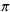Create a new printableGeometry
Math Worksheets

Sample - Click above to make a new math worksheet (PDF).
 Name _____________________________Date ___________________
Perimeter and Area
Find the circumference and area of each circle. State your answer in terms ofand also round your answer to the nearest tenth.

1.

2.
 diameter = 21.56 mm

3.

4.
 diameter = 50.46 cm

5.
 911
cm

6.
 diameter = 44.38 mm

7.
 diameter = 14.88 m

8.

9.
 23
m

 10 * This is a pre-made sheet.Use the link at the top of the page for a printable page.
11.

12.
 diameter = 50 mm

13.
 diameter = 8 cm

14.
 34
m

15.
 diameter = 4.52 mm

16.

17.
 diameter = 16 mm

18.

 19 * This is a pre-made sheet.Use the link at the top of the page for a printable page.
20.
 diameter = 26 m

21.

22.

23.
diameter =
 411
cm

24.

25.
 diameter = 34 mm

26.

27.
 diameter = 36 m